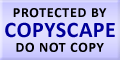Submit Assignment From Here
Name
:
Email
:
Phone
:
Country
:
Willing to pay US\$
:
Subject
:
Topic
:
Levels
:
:Hours
:

One-Sample Hypothesis Test Homework Help

Hypothesis is a way developed for testing the value of the parameters of population. Examples of hypothesis from population point of view are, mean monthly expenditure of departmental store keepers on the supplies.
The testing of hypothesis is based on sample proofs and probability, which are used as tools to determine whether hypothesis is a reasonable statement that will be accepted and not rejected, or is an unreasonable statement which should not be accepted and rejected. Hypothesis tests are conducted in pairs. There are 2 types of hypothesis, alternate and null. Alternate hypothesis is the objective the user is trying to establish and null hypothesis is the objective that user wants to reject or disapprove.
Hypothesis testing is performed in following ways:
a) Firstly we have to state the null and alternate hypothesis.
b) We have to state the significance level and the sample size we have want to use.
c) State the form of statistical form to be used.
d) Apply decision rules based on step c) above.
e) Select samples, collect data and calculate the value of test and compare the p – value.
f) Finally user has to decide by making a comparison among the p – value and significance level of the test.

The figure below is a part of hypothesis testing distribution:Make Payment OnlineUS